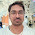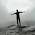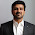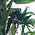Custom UDF in Apache Spark

Apache Spark has become very widely used framework to build Big data application. Spark SQL has made adhoc analysis on structured data very easy, So it is very popular among users who deal with huge amount of structured data. However many times you will realise that some functionality is not available in Spark SQL. For example in Spark 1.3.1 , when you are using sqlContext basic functions like variance , standard deviation and percentile are not available(You can access these using HiveContext). In a similar situation, you may like to do some string operation on an input column but functionality may not be available in sqlContext. In such situations , we can use spark udf to add new constructs in sqlContext. I have found them very handy, specially while processing string type columns. In this post we will discuss how to create UDFs in Spark and  how to use them.

Use Case : Let us keep this example very simple. We will create a udf which will take a string as input and it will convert it into upper case. we will take an example to use that udf with Data Frame.

Create a UDF :  First of all let us see how to declare a UDF. Following is the syntax to create a UDF in spark using scala as language.

import org.apache.spark.sql._
import org.apache.spark.sql.functions._
val toUpper = udf[String, String]( x => x.toUpperCase() )

As you can see it is very simple to define a UDF.
Here first two lines are just imports. we are importing the packages that are required by piece of code that we are writing. Third line is the place where UDF is created. Note the following things in code.
1. toUpper is the name of UDF
2. Notice udf[String, String] part. first argument is type of value that will be returned by this UDF. second argument is type of input argument. If there are multiple input arguments, we have to mention their type in similar way. For example if we are creating a sum udf , which will take two int as input and return int value. then its definition will be as following

val getSum = udf[Int, Int, Int]( x,y => x + y )

Using UDF : Once UDF is created, we can use  it in our code. suppose we have a spark dataframe named df. df has a column named name which contains name. Type of name is String. we want to add one more column in our dataframe with name name_upper where name will be in upper case. Now in this case we can use our udf that we defined earlier.

val df_new = df.withColumn("name_upper", toUpper(df.col("name")))

As you can see that once we create a UDF, using them is very convenient.

Add on : some times when we are working on data frame , we want to add a new column with some dummy value. Now UDFs can be very handy in this case. you can do this using following.
Create a dummy UDF.

val dummy= udf[String,String] (x => x)

Now this udf can be called to add a dummy value column in dataframe.

val df_new = df.withColumn("dummy_col", dummy(lit("Dummy_value")))

here is video on same topic

1.Short and sweet... Good things for daily task.. Thanks for sharing Harjeet!

1.Thanks Devendra :)

2.Simple yet powerful tutorial! UDFs can be life-savers many times. Thanks for the share.

1.Thanks Aswin :)

3.1.Thanks Rao :)

4.hi i want to take two columns as input and perform aggregrations on top of and to get results

val effeciency_func = udf((col1: Double, col2: Double) => {
|
| if (col1 == 0)
| {
| 0
| }
| else {
|
| col2/col1
|
| } })
effeciency_func: org.apache.spark.sql.expressions.UserDefinedFunction = UserDefinedFunction(,DoubleType,Some(List(DoubleType, DoubleType)))

But when i am using that function i get following error.

val sql = "select op_codes as col1,col2 as hrs, col3 as t_hrs, effeciency_func(col1,col2) as effe from history_detail"

scala> val df = spark.sql(sql)
org.apache.spark.sql.AnalysisException: Undefined function: 'effeciency_func'. This function is neither a registered temporary function nor a permanent function registered in the database 'default'.; line 1 pos 79